• ## C语言学生信息管理系统

万次阅读 多人点赞 2012-09-17 12:58:15
大一上学期学习了C，学的不好，对C的理解仅限于编写一些简单的小程序，下学期刚开学，老师就要求我们用C编写一个学生信息管理系统，当时就蒙了，后来讲到需要文件操作，更傻了，当时就没怎么学，后来到处查找资料，...
        大一上学期学习了C，学的不好，对C的理解仅限于编写一些简单的小程序，下学期刚开学，老师就要求我们用C编写一个学生信息管理系统，当时就蒙了，后来讲到需要文件操作，更傻了，当时就没怎么学，后来到处查找资料，向同学，老师请教，慢慢的从能够编写简单的文件写入，然后一步步将每一个函数实现，实现对其的写入，浏览，查询，计算，修改，最后完成删除。后面不断的对代码进行完善，到现在还有很多不足之处，需要和大家一同学习。
上图：代码：

#include<stdio.h>
#include<stdlib.h>
#include<string.h>
typedef struct person                               //定义结构体
{
char num;   //学号
char name; //姓名
int age;      //年龄
int en;      //英语成绩
int ji;      //专业成绩
}person;
person per;  //定义100
char filename;                                      //定义全局变量
logon()                                            //登陆函数 使系统更显完善
{
printf("\n\n\n\t\t\t  学生信息管理系统\n\n");
printf("\t\t\t     版本号：2.0\n\n");
printf("\n\n\n\n\t\t\t    2012年3月10日\n\n");
printf("\t\t\t   C区**栋514寝\n");
printf("\n\n\t\t\t     Ace_cm_liu\n");
printf("\n\n\t\t\t    导演兼制作\n");
system("pause");
}
{
system("cls");
printf("请输入你要操作的文件名:");
scanf("\t%s",filename);
}
//*********************************************************************//
//^^^^^^^^^^^^^^^^^^^^^^^^^^^^^^^^^^^^^^^^^^^^^^^^^^^^^^^^^^^^^^^^^^^^^//
{
char c;
do{
system("cls");
printf("\t\t ╭═════════■□■□═══╮\n");
printf("\t\t│         学生信息管理系统         │\n");
printf("\t\t╰═══■□■□══════════╯\n");
printf("\t\t   ┌─────────────—┐\n");
printf("\t\t   │ 1. 添加记录    2. 显示记录 │\n");
printf("\t\t   │                            │\n");
printf("\t\t   │ 3. 信息查询    4. 数据计算 │\n");
printf("\t\t   │                            │\n");
printf("\t\t   │ 5. 修改记录    6. 删除记录 │\n");
printf("\t\t   │                            │\n");
printf("\t\t   │ 0. 退出程序                │\n");
printf("\t\t   └─────────────—┘\n");
printf("\t\t请您选择(0-6):");
c=getchar();
}while(c<'0'||c>'6');
return (c-'0');                                      //字符减去’0‘得到的是数字整型//
}

//*********************************************************************//
//^^^^^^^^^^^^^^^^^^^^^^^^^^^^^^^^^^^^^^^^^^^^^^^^^^^^^^^^^^^^^^^^^^^^^//

{
FILE *fp;
int i=0,n=0;
char sign='y';                              //首先定义sing='y'
system("cls");                                //清屏函数  系统中调用
while(sign!='n'&&sign!='N')                    //其实只有当输入N或n时才跳出循环
{
printf("             ----学生纪录添加----\n");
printf("学号：\n");
scanf("\t%s",per[i].num);
printf("姓名:\n");
scanf("\t%s",per[i].name);
printf("年龄：\n");
scanf("\t%d",&per[i].age);
printf("英语：\n");
scanf("\t%d",&per[i].en);
printf("专业：\n");
scanf("\t%d",&per[i].ji);
printf("是否继续添加?(y/n)");
scanf("\t%c",&sign);
i++;
n=n+1;
system( "cls ");
}system("pause");                                     //等待命令

if((fp=fopen(filename,"a"))==NULL)                    //以2进制形式进行文件尾追加//
{
printf("cannot open the files\n");
system("pause");
return;                                         //如果文件出现错误 就返回
}
for(i=0;i<n;i++)
if(fwrite(&per[i],sizeof(person),1,fp)!=1)      //以2进制形式写入文件中//一次写入一个数组//
printf("file write error\n");
fclose(fp);                                       //关闭文件
system("pasue");
}
//*********************************************************************//
//^^^^^^^^^^^^^^^^^^^^^^^^^^^^^^^^^^^^^^^^^^^^^^^^^^^^^^^^^^^^^^^^^^^^^//
void scan()                        //显示记录  进行全面信息浏览
{
void amend();                         //声明修改函数//
char sign='y';
FILE *fp;
int i=0,n;
system("cls");
if((fp=fopen(filename,"r"))==NULL)             //以2进制方式读取文件信息//
{
printf("cannot open the files\n");
system("pause");
return;
}
while(!feof(fp))                              //一直读取知道文件尾结束
{
i++;
}
fclose(fp);
printf("^^^^^^^^^^^^^^^^^^^^^^^^^^^^^^^^^^^^^^^^^^^^^^^^^^^^^^^^^^^^\n");
printf("        学号            姓名           年龄    英语    专业\n");
printf("^^^^^^^^^^^^^^^^^^^^^^^^^^^^^^^^^^^^^^^^^^^^^^^^^^^^^^^^^^^^\n");
for(n=0;n<i-1;n++)                             //循环全部输出
printf("\t%s \t%s \t\t%d \t%d \t%d\n",per[n].num,per[n].name,per[n].age,per[n].en,per[n].ji);
printf("^^^^^^^^^^^^^^^^^^^^^^^^^^^^^^^^^^^^^^^^^^^^^^^^^^^^^^^^^^^^\n");
system("pause");
system("cls");
printf("是否需要修改?(y/n)");
scanf("\t%c",&sign);
if(sign!='n'&&sign!='N')                    //选择是否在浏览后进行修改//
amend();
system("pause");

}

//*********************************************************************//
//^^^^^^^^^^^^^^^^^^^^^^^^^^^^^^^^^^^^^^^^^^^^^^^^^^^^^^^^^^^^^^^^^^^^^//
//数据查询//
void query()                     //学生信息的不同方式查询
{
FILE *fp;
int i=0,n=0;
int m;
system("cls");
printf("\t\n请选择查询方式:\n");
printf("\t┌──────┐\n");
printf("\t│1--模糊查询 │\n");
printf("\t│2------姓名 │\n");
printf("\t│3------学号 │\n");
printf("\t│4------返回 │\n");
printf("\t└──────┘\n");
printf("请输入你要查询的方式\n");
scanf("%d",&m);
while(m!=1&&m!=2&&m!=3&&m!=4)
{
printf("输入错误请重新查询\n");
scanf("%d",&m);
}
if((fp=fopen(filename,"r"))==NULL)                 //以2进制方式读取文件信息//
{
printf("cannot open the files\n");
system("pause");
return;
}

while(!feof(fp))
{
i++;n=n+1;
}

if(m==1)                                                 //模糊查询
{
char s;                                             //定义输入的文字所占的字节 /一个汉字占2个字节 外加一结尾符此处小心溢出
int i=0;
system("cls");
printf("\t请输入想查询的姓:");
scanf("%s",s);
for(i=0;i<n-1;i++)                                               //文件信息已经读取到数组中 此处进行循环用于下一语句的查找
{

if(per[i].name==s&&per[i].name==s)              //比较per[i].name与所输入的前俩个字符是否相同//
{
printf("\t学号: %s\n",per[i].num);
printf("\t姓名: %s\n",per[i].name);
printf("\t年龄: %d\n",per[i].age);
printf("\t英语: %d\n",per[i].en);
printf("\t计算机: %d\n",per[i].ji);
printf("\t                     \n");
}
}
}

if(m==2)                                            //姓名查询
{
char s;
int i=0;
printf("\t请输入想查询的姓名:");
scanf("\t%s",s);
while(strcmp(per[i].name,s)!=0&&i<n) i++;            //比较per[i].name与所输入姓名是否相同//
if(i==n)
{
printf("\t通讯录中没有此人!\n");
system("pause");
return;
}
printf("\t学号: %s\n",per[i].num);
printf("\t姓名: %s\n",per[i].name);
printf("\t年龄: %d\n",per[i].age);
printf("\t英语: %d\n",per[i].en);
printf("\t计算机: %d\n",per[i].ji);
}
if(m==3)                                                            //学号查询
{
char s;
int i=0;
printf("请输入想查询的学号：");
scanf("\t%s",s);
while(strcmp(per[i].num,s)!=0&&i<n)i++;                       //比较per[i].name与所输入学号是否相同//
if(i==n)
{
printf("\t通讯录中没有此人!\n");
system("pause");
return;
}
printf("\t学号: %s\n",per[i].num);
printf("\t姓名: %s\n",per[i].name);
printf("\t年龄: %d\n",per[i].age);
printf("\t英语: %d\n",per[i].en);
printf("\t计算机: %d\n",per[i].ji);
}
fclose(fp);
system("pause");
}
//*********************************************************************//
//^^^^^^^^^^^^^^^^^^^^^^^^^^^^^^^^^^^^^^^^^^^^^^^^^^^^^^^^^^^^^^^^^^^^^//
//数据统计//
void date()
{
FILE *fp;
struct person temp;                               //定义临时数组用于交换时存放数组信息用于后面的交换//
int sum;                                         //总分
int s;
int i=0,n=0;
int j,k,t;
int m;
system("cls");
printf("\t\n选择排序方式:\n");
printf("\t┌──────┐\n");
printf("\t│1------英语 │\n");
printf("\t│2----计算机 │\n");
printf("\t│3----  总分 │\n");
printf("\t│4------返回 │\n");
printf("\t└──────┘\n");
printf("请输入你要排序的方式\n");
scanf("%d",&m);
system("cls");
while(m!=1&&m!=2&&m!=3&&m!=4)
{
printf("输入错误请重新输入\n");
scanf("%d",&m);
}
if((fp=fopen(filename,"r"))==NULL)                 //以2进制方式读取文件信息//
{
printf("cannot open the files\n");
system("pause");
return;
}

while(!feof(fp))                               //一直进行读取直至文件尾//
{
i++;n=n+1;
}
if(m==1)
{
for(i=0;i<n-1;i++)                           //采用冒泡法进行排序//
{
k=i;
for(j=i+1;j<n;j++)
if(per[j].en>per[k].en)                              //排序//比较大小//
k=j;
temp=per[k];per[k]=per[i];per[i]=temp;                  //当符合条件时进行数据交换//
}
printf("^^^^^^^^^^^^^^^^^^^^^^^^^^^^^^^^^^^^^^^^^^^^^^^^^^^^^^^^^^^^\n");
printf("        学号            姓名           年龄    英语    专业\n");
printf("^^^^^^^^^^^^^^^^^^^^^^^^^^^^^^^^^^^^^^^^^^^^^^^^^^^^^^^^^^^^\n");
for(i=0;i<n-1;i++)
printf("\t%s \t%s \t\t%d \t%d \t%d\n",per[i].num,per[i].name,per[i].age,per[i].en,per[i].ji);
printf("^^^^^^^^^^^^^^^^^^^^^^^^^^^^^^^^^^^^^^^^^^^^^^^^^^^^^^^^^^^^\n");
fclose(fp);
}

if(m==2)
{
for(i=0;i<n-1;i++)
{
k=i;
for(j=i+1;j<n;j++)
if(per[j].ji>per[k].ji)
k=j;
temp=per[k];per[k]=per[i];per[i]=temp;
}
printf("^^^^^^^^^^^^^^^^^^^^^^^^^^^^^^^^^^^^^^^^^^^^^^^^^^^^^^^^^^^^\n");
printf("        学号            姓名           年龄    英语    专业\n");
printf("^^^^^^^^^^^^^^^^^^^^^^^^^^^^^^^^^^^^^^^^^^^^^^^^^^^^^^^^^^^^\n");
for(i=0;i<n-1;i++)
printf("\t%s \t%s \t\t%d \t%d \t%d\n",per[i].num,per[i].name,per[i].age,per[i].en,per[i].ji);
printf("^^^^^^^^^^^^^^^^^^^^^^^^^^^^^^^^^^^^^^^^^^^^^^^^^^^^^^^^^^^^\n");
fclose(fp);
}
if(m==3)
{
for(i=0;i<n-1;i++)
{
sum=0;
sum=per[i].en+per[i].ji;
s[i]=sum;                                           // 第一个数组用于数组间信息操作//
}
for(i=0;i<n-1;i++)
{
k=i;
for(j=i+1;j<n;j++)
if(s[j]>s[k])
k=j;
t=s[k];s[k]=s[i];s[i]=t;                          //按照总分大小进行排序//
temp=per[k];per[k]=per[i];per[i]=temp;            //随之使其数组相应顺序随总分大小一起排序//
}
printf("^^^^^^^^^^^^^^^^^^^^^^^^^^^^^^^^^^^^^^^^^^^^^^^^^^^^^^^^^^^^^^^^^^^^^\n");
printf("        学号            姓名           年龄    英语    专业    总分   \n");
printf("^^^^^^^^^^^^^^^^^^^^^^^^^^^^^^^^^^^^^^^^^^^^^^^^^^^^^^^^^^^^^^^^^^^^^\n");
for(i=0;i<n-1;i++)
printf("\t%s \t%s \t\t%d \t%d \t%d \t%d\n",per[i].num,per[i].name,per[i].age,per[i].en,per[i].ji,s[i]);
printf("^^^^^^^^^^^^^^^^^^^^^^^^^^^^^^^^^^^^^^^^^^^^^^^^^^^^^^^^^^^^^^^^^^^^^\n");
fclose(fp);
}
system("pause");
}

//*********************************************************************//
//^^^^^^^^^^^^^^^^^^^^^^^^^^^^^^^^^^^^^^^^^^^^^^^^^^^^^^^^^^^^^^^^^^^^^//
// 数据修改//
void amend()
{
FILE *fp;
int i=0,n=0;
char s;
int m;
system("cls");
printf("\t\n请选择修改方式:\n");
printf("\t┌──────┐\n");
printf("\t│1------姓名 │\n");
printf("\t│2------学号 │\n");
printf("\t│3------返回 │\n");
printf("\t└──────┘\n");
printf("请输入你要修改的方式\n");
scanf("%d",&m);
while(m!=1&&m!=2&&m!=3)
{
printf("输入错误请重新修改\n");
scanf("%d",&m);
}
if((fp=fopen(filename,"r"))==NULL)                 //以2进制方式读取文件信息//
{
printf("cannot open the files\n");
system("pause");
return;
}
while(!feof(fp))
{
i++;n=n+1;
}i=0;
fclose(fp);
system("cls");
if(m==1)
{
char s;
int i=0;
printf("\t请输入想修改的姓名:");
scanf("\t%s",s);
while(strcmp(per[i].name,s)!=0&&i<n) i++;                           //进行比较 找到所需要的信息
if(i==n)
{
printf("\t通讯录中没有此人!\n");
return;
}
else{
printf("学号：\n");
scanf("\t%s",per[i].num);
printf("姓名:\n");
scanf("\t%s",per[i].name);
printf("年龄：\n");
scanf("\t%d",&per[i].age);
printf("英语：\n");
scanf("\t%d",&per[i].en);
printf("专业：\n");
scanf("\t%d",&per[i].ji);
}
}
if(m==2)
{
char s;
int i=0;
printf("请输入想修改的学号：");
scanf("\t%s",s);
while(strcmp(per[i].num,s)!=0&&i<n)i++;
if(i==n)
{
printf("\t通讯录中没有此人!\n");
return;
}
else{
printf("学号：\n");
scanf("\t%s",per[i].num);
printf("姓名:\n");
scanf("\t%s",per[i].name);
printf("年龄：\n");
scanf("\t%d",&per[i].age);
printf("英语：\n");
scanf("\t%d",&per[i].en);
printf("专业：\n");
scanf("\t%d",&per[i].ji);
}
}
if((fp=fopen(filename,"w"))==NULL)                            //进行修改后将信心重新写入文件中
{
printf("cannot open the files\n");
return;
}
for(i=0;i<n-1;i++)
if(fwrite(&per[i],sizeof(person),1,fp)!=1)
printf("file write error\n");
fclose(fp);
printf("信息已经修改成功\n");
system("pause");
}
//*********************************************************************//
//^^^^^^^^^^^^^^^^^^^^^^^^^^^^^^^^^^^^^^^^^^^^^^^^^^^^^^^^^^^^^^^^^^^^^//
//数据删除//
void delet()
{
FILE *fp;
char s;
int i=0,n=0;
if((fp=fopen(filename,"r"))==NULL)                  //以2进制方式读取文件信息//
{
printf("cannot open the files\n");
system("pause");
return;
}

while(!feof(fp))
{
i++;n=n+1;
}
fclose(fp);
if((fp=fopen(filename,"w"))==NULL)                 //以2进制写入方式打开文件//
{
printf("cannot open the files\n");
system("pause");
return;
}
printf("学生学号：");
scanf("%s",s);
for(i=0;i<n-1;i++)
if(!(strcmp(per[i].num,s)==0))                    //找到与其匹配的信息进行操作//   如果条件符合就写入否者跳过这组数据此数据就是所需删除的
fwrite(&per[i],sizeof(person),1,fp)!=1;             //以2进制方式将信息从内存中写入文件磁盘//
fclose(fp);
printf("数据删除成功");
system("pause");
}
//^^^^^^^^^^^^^^^^^^^^^^^^^^^^^^^^^^^^^^^^^^^^^^^^^^^^^^^^^^^^^^^^^^^^^//
//^^^^^^^^^^^^^^^^^^^^^^^^^^^^^^^^^^^^^^^^^^^^^^^^^^^^^^^^^^^^^^^^^^^^^//
//运用switch语句进行菜单选择//
void main()
{
int n=0;
logon();
for(;;)                                                 //循环使用主界面直到遇到结束命令
{
{
case 1:
printf("\t\t *^_^*学生记录添加 *^_^*\n");
break;
case 2:
printf("\t\t *^_^*学生信息浏览 *^_^*\n");
scan();
break;
case 3:
printf("\t\t *^_^*学生信息查询 *^_^*\n");
query();
break;
case 4:
printf("\t\t *^_^*数据计算 *^_^*\n");
date();
break;
case 5:
printf("\t\t *^_^*数据修改 *^_^*\n");
amend();
break;
case 6:
printf("\t\t *^_^*数据删除 *^_^*\n");
delet();
break;
case 0:
printf("\n\t\t谢谢使用，再见!\n");
printf("\n\t\t");
system("pause");
exit(0);
}
}
system("pause");
}

//学生信息管理系统前期功程完成，还有待修改使其功能更加完善代码更加简洁巧妙  thank you!//
// 如果这段代码好用，那它是Ace_cm_liu写的。
//如果不好用，我不知道是谁写的。


展开全文c 语言 system fp null
• c语言项目管理系统 c语言初学者 简单c语言 学籍银行管理系统
• ## C语言学生管理系统

千次阅读 多人点赞 2019-05-08 10:54:10
系统实现对一个班学生成绩的管理功能包括： 1 新增学生信息 2 查看学生信息 3 修改学生信息 4 删除学生信息 5 打印成绩单 代码实现如下： /* *Study Code *language:C/C++ *author:lzm */ #...
本系统实现对一个班学生成绩的管理功能包括：1 新增学生信息，2 查看学生信息，3 修改学生信息，4 删除学生信息，5 打印成绩单。
代码以模块化设计形式，每一个模块都有独立的功能，代码采用C/C++编写，代码中包含了一些对于萌新来说新的知识，比如说成绩排序用到了C语言的内置排序函数qsort()，而且还是结构体二级排序，此外还用到的“引用”用法，这些都将需要你去学习，一些好的方法需要掌握，好了，为了代码易懂，我还是写的比较清楚的，没有在代码上做细节处理，就是按照正常的思路来写，便于阅读，不像有些作者炫耀自己的水平往往加入以下让人难以理解的代码，废话不啰嗦了，代码设计如下：
欢迎加入C语言学习交流QQ群:261503823
欢迎使用在线代码测试平台:www.iuacc.cn
包含头文件及宏
/*
*Study Code
*language:C/C++
*author:lzm
*/

#include <stdio.h>
#include <string.h>
#include <stdlib.h>
#define MAX_STD 1005

定义学生结构体
/**
*单个学生信息结构体
*/
typedef struct STD
{
char number;/**学号*/
char name;	/**姓名*/
double score;/**3门课成绩*/
int rank;	/**名次*/

}Std;

定义班级结构体
/**
*一个班级的学生信息
*/
typedef struct STD_CLASS
{
Std Student[MAX_STD];/**记录一个班所有学生的信息*/
int StudentCount; /**记录一个班级学生的个数*/

}Std_Class;

班级初始化
/**
*初始化班级信息
*/
void initStdClass(Std_Class &std_class)/**使用引用，详情百度引用的使用方法*/
{
std_class.StudentCount = 0;/**一开始班级学生数为0*/
}

新增一名学生信息
/**
*新增一名学生
*/
{
int index = std_class.StudentCount;//获取当前的班级学生个数
printf("添加:\n");
printf("请输入新学号:");
scanf("%s",std_class.Student[index].number);
printf("请输入新姓名:");
scanf("%s",std_class.Student[index].name);
printf("请输入成绩1:");
scanf("%lf",&std_class.Student[index].score);
printf("请输入成绩2:");
scanf("%lf",&std_class.Student[index].score);
printf("请输入成绩3:");
scanf("%lf",&std_class.Student[index].score);
printf("添加完成!\n");
std_class.StudentCount++;//学生数加1
}

查看学生信息
/**
*查看一名学生信息
*以学号查找
*/
void findStudent(Std_Class &std_class)
{
if(std_class.StudentCount < 1)//班级没有学生
{
printf("暂无学生,请添加!\n");
return ;
}
printf("查看:\n");
printf("请输入学号:");
char number;
scanf("%s",number);
for(int i=0;i<std_class.StudentCount;i++)
{
if(strcmp(std_class.Student[i].number,number)  ==  0)//找到查看的学生
{
printf("----------------------------------------------------\n");
printf("学号\t姓名\t成绩1\t成绩2\t成绩3\t总分\t排名\n");
printf("----------------------------------------------------\n");
printf("%s\t",std_class.Student[i].number);//打印学号
printf("%s\t",std_class.Student[i].name);//打印姓名
printf("%.f\t",std_class.Student[i].score);//打印成绩1
printf("%.f\t",std_class.Student[i].score);//打印成绩2
printf("%.f\t",std_class.Student[i].score);//打印成绩3
printf("%.f\t",std_class.Student[i].score + std_class.Student[i].score +std_class.Student[i].score);//总成绩
printf("%d\n",std_class.Student[i].rank);
printf("----------------------------------------------------\n");
return ;
}
}
printf("查无此学号!\n");
return ;
}

修改学生信息
/**
*修改一名学生信息
*以学号查找
*/
void reviseStudent(Std_Class &std_class)
{
if(std_class.StudentCount < 1)//班级没有学生
{
printf("暂无学生,请添加!\n");
return ;
}
printf("修改:\n");
printf("请输入学号:");
char number;
scanf("%s",number);
for(int i=0;i<std_class.StudentCount;i++)
{
if(strcmp(std_class.Student[i].number,number) == 0)
{
while(1)
{
printf("\t-----修改-----\n");
printf("\t1 修改姓名\n");
printf("\t2 修改成绩1\n");
printf("\t3 修改成绩2\n");
printf("\t4 修改成绩3\n");
printf("\tQ 退出修改\n");
char op;
printf("请输入:");
scanf("%s",op);
int len = strlen(op);
if(op >= '1' && op <= '5'&& len == 1)//输入操作数合法
{
if(op == '1')//修改姓名
{
printf("请输入新姓名:");
scanf("%s",std_class.Student[i].name);
}
else if(op == '2')//修改成绩1
{
printf("请输入成绩1:");
scanf("%lf",&std_class.Student[i].score);
}
else if(op == '3')//修改成绩2
{
printf("请输入成绩2:");
scanf("%lf",&std_class.Student[i].score);
}
else if(op == '4')//修改成绩3
{
printf("请输入成绩3:");
scanf("%lf",&std_class.Student[i].score);
}
printf("修改成功!\n");
}
else if(op == 'Q' && len == 1 )//退出修改
{
return;
}
else //输入操作数不合法
{
printf("您的输入有误,请重新输入:\n");
}
}
}
}
printf("查无此学号!\n");
return ;
}

删除学生信息
/**
*删除一名学生信息
*以学号删除
*/
void deleteStudent(Std_Class &std_class)
{
if(std_class.StudentCount < 1)//班级没有学生
{
printf("暂无学生,请添加!\n");
return ;
}
printf("删除:\n");
printf("请输入学号:");
char number;
scanf("%s",number);
for(int i=0;i<std_class.StudentCount;i++)
{
if(strcmp(std_class.Student[i].number,number) == 0)//找到将要删除的学号
{
for(int j=i;j<std_class.StudentCount-1;j++)//向前覆盖
{
strcpy(std_class.Student[j].number,std_class.Student[j+1].number);
strcpy(std_class.Student[j].name,std_class.Student[j+1].name);
std_class.Student[j].rank = std_class.Student[j+1].rank;
std_class.Student[j].score = std_class.Student[j+1].score;
std_class.Student[j].score = std_class.Student[j+1].score;
std_class.Student[j].score = std_class.Student[j+1].score;
}
printf("删除成功!\n");
std_class.StudentCount--;
return ;
}
}
printf("查无此学号!\n");
return ;
}

对学生成绩排序
/**
*二级排序
*/
int cmp(const void *a,const void *b)
{
struct STD *c = (STD *)a;
struct STD *d = (STD *)b;
double a_score = c->score + c->score + c->score;
double b_score = d->score + d->score + d->score;
if(a_score == b_score)
{
return strcmp(c->number,d->number);
}
return a_score < b_score;
}
/**
*对学生信息排序
*按照总成绩排序
*总成绩相同按照学号排序
*/
void rankStudent(Std_Class &std_class)
{
/**对结构体排序，C语言内置排序函数*/
/**https://blog.csdn.net/yisandezhuiqiu/article/details/52101667*/
qsort(std_class.Student,std_class.StudentCount,sizeof(std_class.Student),cmp);//对结构体排序
for(int i=0;i<std_class.StudentCount;i++)
{
std_class.Student[i].rank = i+1;//设置名次
}
}

打印成绩单
/**
*打印成绩单
*/
void printStudent(Std_Class &std_class)
{
printf("成绩单:\n");
printf("----------------------------------------------------\n");
printf("学号\t姓名\t成绩1\t成绩2\t成绩3\t总分\t排名\n");
printf("----------------------------------------------------\n");
if(std_class.StudentCount < 1)
{
printf("\t\t  暂无学生,请添加!\n");
printf("----------------------------------------------------\n");
return ;
}
for(int i=0;i<std_class.StudentCount;i++)
{
printf("%s\t",std_class.Student[i].number);
printf("%s\t",std_class.Student[i].name);
printf("%.f\t",std_class.Student[i].score);
printf("%.f\t",std_class.Student[i].score);
printf("%.f\t",std_class.Student[i].score);
printf("%.f\t",std_class.Student[i].score + std_class.Student[i].score + std_class.Student[i].score);
printf("%d\n",std_class.Student[i].rank);
printf("----------------------------------------------------\n");
}
return ;
}

主菜单
int Menu()
{
printf("\t-----菜单-----\n");
printf("\t1 添加学生信息\n");
printf("\t2 查看学生信息\n");
printf("\t3 修改学生信息\n");
printf("\t4 删除学生信息\n");
printf("\t5 打印成绩单\n");
printf("\tQ 退出\n");
char op;
printf("请输入:");
while(scanf("%s",op)!=EOF)
{
int len = strlen(op);
if(op >= '1' && op <= '5'&& len == 1)//合法的操作数
{
return (int)(op - '0');//返回整数 1-5
}
else if(op == 'Q' && len == 1 )
{
return 0;
}
else //输入操作数不合法
{
printf("您的输入有误,请重新输入:");
}
}
}

主程序
int main()
{
Std_Class OneClass;/**一班*/
initStdClass(OneClass);//初始化

while(1)
{
if(op == 1)//添加
{
rankStudent(OneClass);//重新排序
}
else if (op == 2)//查看
{
findStudent(OneClass);//查看学生信息
}
else if (op == 3)//修改
{
reviseStudent(OneClass);//修改学生信息
rankStudent(OneClass);//重新排序
}
else if (op == 4)//删除
{
deleteStudent(OneClass);//删除学生信息
rankStudent(OneClass);//重新排序
}
else if(op == 5)
{
printStudent(OneClass);//打印成绩单
}
else if(op == 0)
{
break;//结束
}
}
return 0;
}

运行结果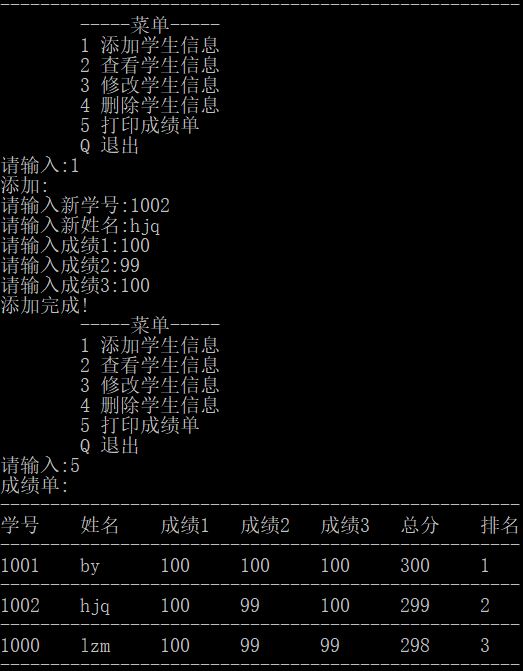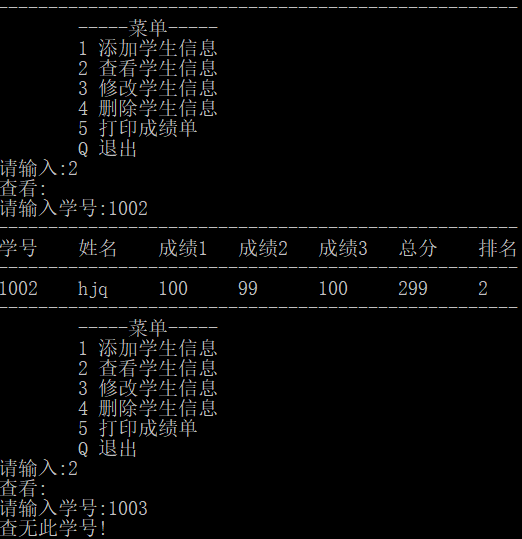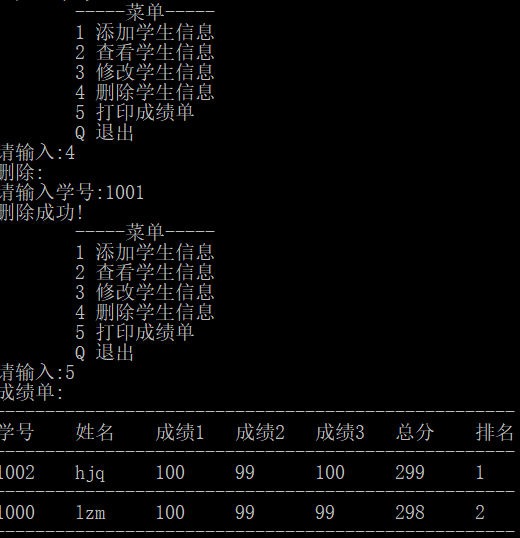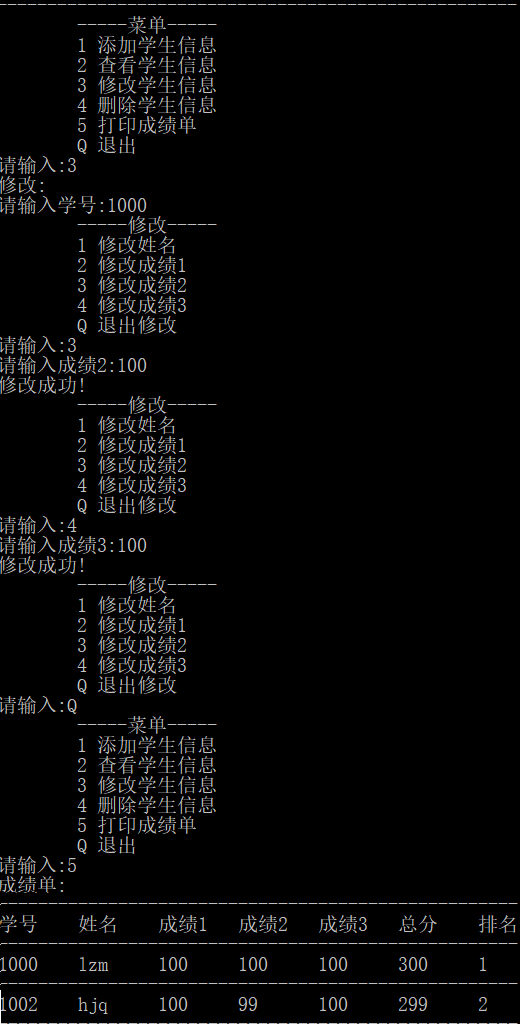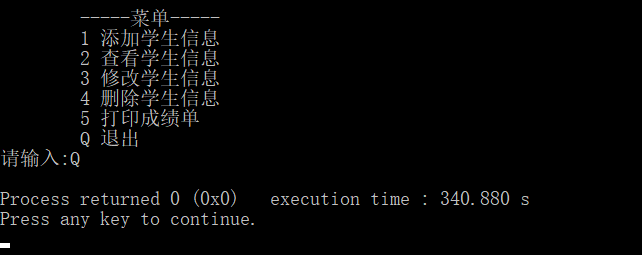以上代码仅供学习参考。
欢迎加入C语言学习交流QQ群:261503823
欢迎使用在线代码测试平台:www.iuacc.cn


展开全文• C语言实现中小型酒店旅馆管理系统 刚刚基础的学完一遍c语言，在老师的带领下，大致完成了这个项目，其中因为时间问题，有些地方还未修改，功能有点不完善。虽然做的过程，学c语言的过程很痛苦，想过放弃，但是坚持...
C语言实现中小型酒店旅馆管理系统
刚刚基础的学完一遍c语言，在老师的带领下，大致完成了这个项目，其中因为时间问题，有些地方还未修改，功能有点不完善。虽然做的过程，学c语言的过程很痛苦，想过放弃，但是坚持下来真的学到不少东西，很感谢老师，写点文字以供今后复习回忆。
一.系统的功能需求
1.系统分四种角色分别是： 系统管理员；经理；前台接待员；保洁员；旅客。五个角色对应五个模块，每个模块都有不同的权限。
2.旅客：权限最低，功能：查询房间，查看自己信息。其中查询房间可以显示全部，也可以模糊查询，比如输入“单人”，显示所有类型单人房。
3.除旅客外，其他四个角色统一登录，然后调用对应的子菜单函数。同时他们也有相同的一部分功能，如：修改个人信息（更改账号，密码，姓名，邮箱），注销（退出当前模块）。
4.系统管理员功能：浏览用户，增加用户，删除用户，初始化用户密码，系统备份。系统管理员主要是维护系统，管理用户，备份系统文件。
5.经理功能：浏览用户，增加用户，删除用户，浏览客房，修改客房，增加客房，删除客房，查看收支。经理作为决策者，可以管理自己的员工，根据市场对客房进行增删改，查看每月收入，每间房间的收益，从而完善客房配置，提高旅馆收益。
6.前台接待员功能：查询房间（内分办理入住，办理预约），退房，换房，续住，查看客人信息。前台与旅客接触功能复杂，根据旅客需求选择房间，然后办理入住，预约，填写旅客信息，还有续、换、退房。
7.保洁员功能：查询未打扫房间，确认房间打扫完成。提高旅馆效率，使收益更大化。
8.因为角色较多，功能较多，最后的.c文件会有很多，以及在其他.c文件中也会有很多地方用到另外.c文件中的函数，所以为了方便，创建相应的.h文件，会给我们带来不少的便利。
二.系统的主要模块
1.公共模块

用于实现四种工作人员登录的身份认证，该模块先读取用户信息文件生成带头结点链表，再根据用户输入的账号密码进行匹配，匹配成功返回该节点指针传递给角色调用函数。

2.角色调用函数：void enterSystem(user *r)

接收身份认证模块返回指针，根据信息中的身份调用不同角色函数

3.显示时间界面：void showTime(int k)

根据时间显示上午好，下午好等。

接收身份认证模块返回指针r；对该节点的账号，密码，姓名，邮箱进行修改，但角色，性别不可更改。

5 删除用户函数：void deleteUser()

根据输入账号进行匹配，找到即可删除，否则报错。

6 增加用户函数void write()

按要求输入相关信息，写入用户信息文件中。

7 读取文件信息模块：

8 输出链表模块：
int display_user()输出用户链表
int display_room()输出客房链表
int display_bill()输出账单链表
int display_customer()输出旅客信息链表

9 清除链表模块：清理操作完成后的链表
int delete_user()清除用户链表
int delete_room()清除房间链表

将读取文件函数才是最终形成链表的地方，将它返回的头指针传入输出函数（我是在输出函数中调用读取函数），输出信息即可。清除链表的某一节点后，一定要将那个节点free()掉，同样的添加节点，一定要将新加的节点尾部赋成NULL。
总界面：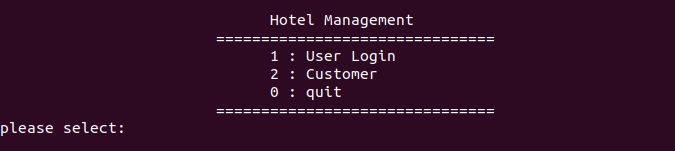2.管理员相关模块

根据输入账号进行匹配，找到即可进行初始化操作，否则报错。
c.备份模块：相关文件的备份。
读取原文件，在写入“原文件名+日期”文件中完成备份
void backupUser()用户文件备份
void backupRoom()客房文件备份
void backupBill()账单文件备份
void backupCustomer()旅客信息文件备份

备份文件的方法
struct tm *newtime;
char outfile;
time_t t1;
t1 = time(NULL);
newtime = localtime(&t1);
strftime(outfile,128,“xxx_%Y%m%d_%H%M%S.txt”,newtime);
这里也是经过网上搜索学到的一种可以把时间保存到文件名的方法，strftime()函数可以将本地时间\日期格式化，将保存的字符串存到相应的字符数组中，%Y%m%d……有很多，可以今后用到查阅。

管理员界面：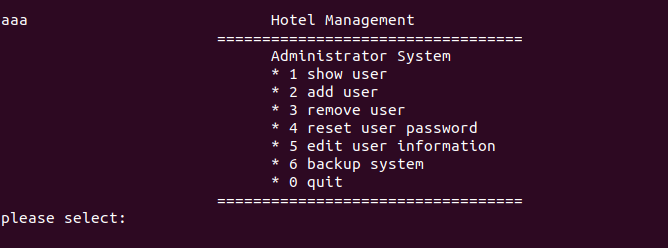3.经理相关模块

a. 经理菜单界面void managerSystem(User *r)
b. 查看账单函数void checkBill()
c.房间操作模块：
void writeRoom()增加房间
void amendRoom()修改房间
void deleteRoom()删除房间

经理界面：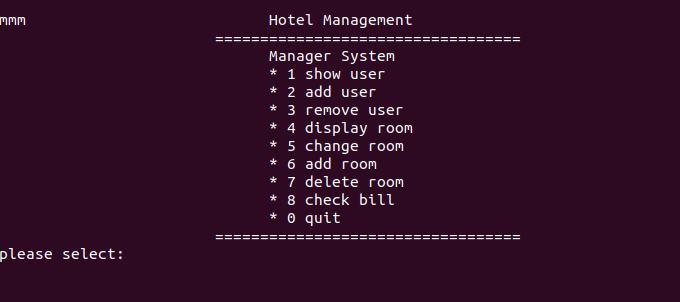经理代码链接：https://gitee.com/hhb845531681/hotel-management/blob/master/src/manager.c

4.前台接待员相关模块

a. 前台接待界面void receptSystem(User *r)
b. void check_stayroom()
查看房间的信息，办理旅客入住，将相应的房间的状态改变并且添加旅客信息到相应的文件。
c. void exit_room()退房函数
输入旅客身份证号，确认旅客信息，从中读取旅客的房间号、入住时间、退房时间，将需要的参数传入相应的函数，将旅客的状态改成已退房，且将退的房的状态改成未打扫。
d. void replace_room()换房函数
换房函数首先应确认现在还有没有可用的房间，确认有后，输入旅客身份证号，确认旅客信息，将原先住的房间状态改成未打扫，将要换的房间的状态改变。
e. void keep_stay()续住函数
输入原房间号，确认旅客信息，在看有无预约（还未完成），输入退房时间，再补交费用，最后更改相应文件信息。
传入入住旅客，房间的信息节点，获得需要的参数，将相关信息写入账单文件

前台接待员界面：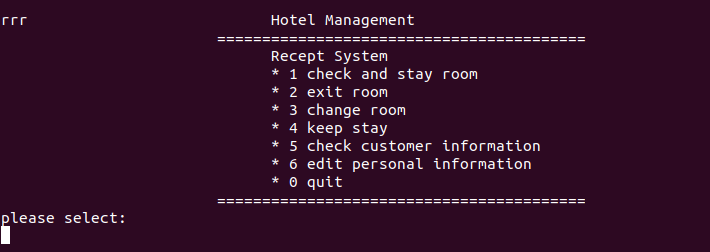前台代码链接：https://gitee.com/hhb845531681/hotel-management/blob/master/src/recept.c

5.保洁员相关模块

a. void cleanerSystem(user *r)保洁菜单界面
b. int cleaner_seeroom()查找未打扫房间
查看房间状态，保洁员确认打扫后将房间状态置为“正在打扫”。
c. int cleanroom()释放房间
保洁员将房间打扫干净后，将房间状态置为可用。

保洁员界面：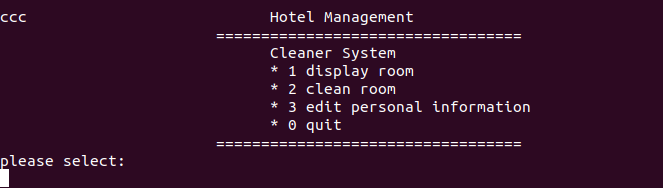保洁员代码链接：https://gitee.com/hhb845531681/hotel-management/blob/master/src/cleaner.c

6.旅客相关模块

a. void customerSystem()游客函数
进入旅客选择菜单
b. int findroom()查找房间
读取房间信息文件，根据选择条件可进行不同类型查找
c. int cusself_informatin()查看自己信息
输入身份证号进行查找，匹配成功输出该节点。

旅客界面：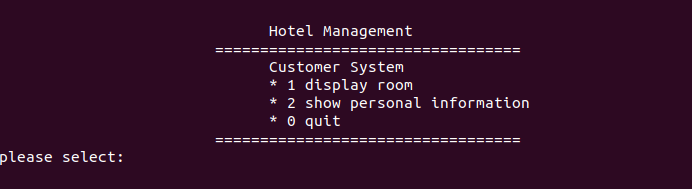旅客代码链接：https://gitee.com/hhb845531681/hotel-management/blob/master/src/customer.c

三.用到的四个结构体

1 . struct usernode
{
char userId;      //工作人员的id号
char name;     //名字
char sex;  //性别
char email;//邮箱
struct usernode *next;
};
typedef struct usernode User;	  //工作人员结构体

2 .struct  roomnode
{
int roomId;//房间号
char type;//房间类型:single(单人间),double(两人间),kingbed(大床房)等等
int price;//房间单价
int discount;//0 normal,1 sale
int cashPledge;//押金
int status;//0 empty,1 not empty,2 not clean,3 cleaning房间四种状态
struct roomnode *next;
};
typedef struct roomnode Room;  //房间结构体

3 .struct  customernode
{
int roomId;//房间号
char cusid;  //旅客身份证
char cusname;//姓名
int cusage;//年龄
char cussex;//性别
char phone;//联系电话
char inDay;//开房时间
char outDay;//退房时间
int status;    //1 .入住  0.以退房  2.预约
struct customernode *next;
};
typedef struct customernode Customer;  //旅客结构体

4 .struct billnode
{
int roomId;//房间号
int price;//房间单价
int yingPrice;//应收金额
int realPrice;  //实收金额
int cashPledge;//押金
int change;//找零
int status;  // 1.入住押金 0.已还押金
char inDay;//开房时间
char outDay;//退房时间
struct billnode *next;
};
typedef struct billnode Bill;//账单结构体

四.部分功能的实现效果
1.工作人员信息陈列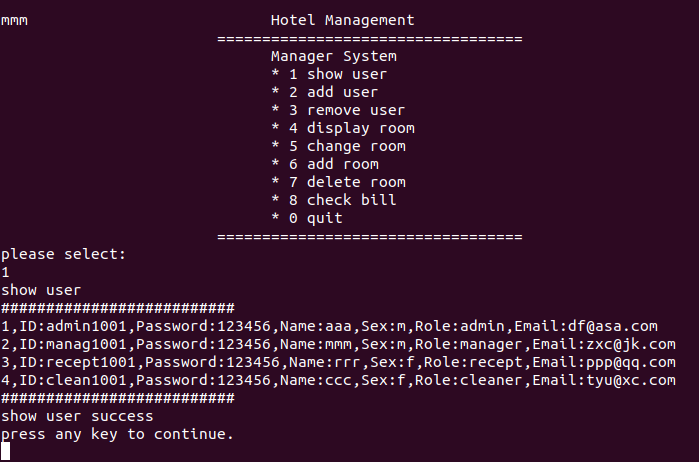2.房间的陈列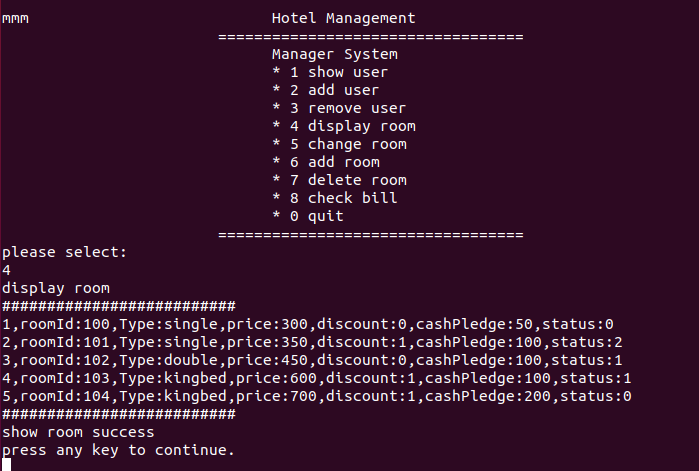3.旅客对房间的选择性查看
只查看单人间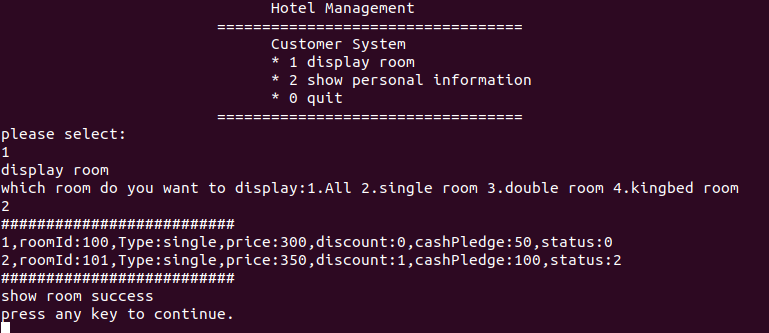只查看两人间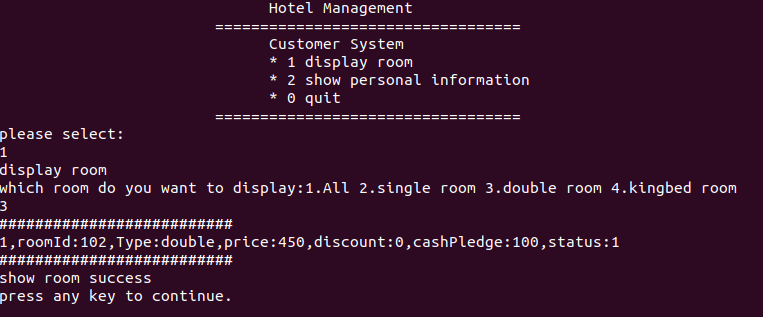只查看大床房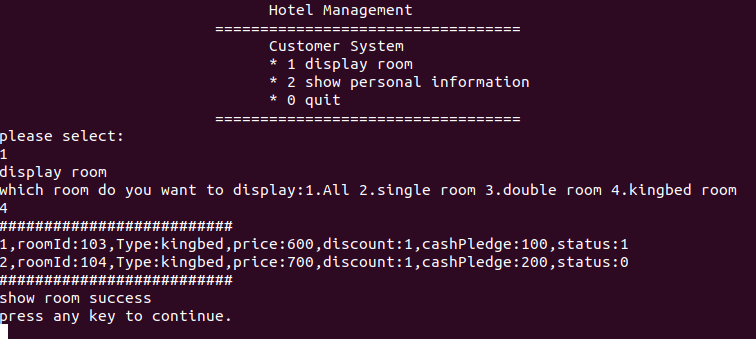4.账单陈列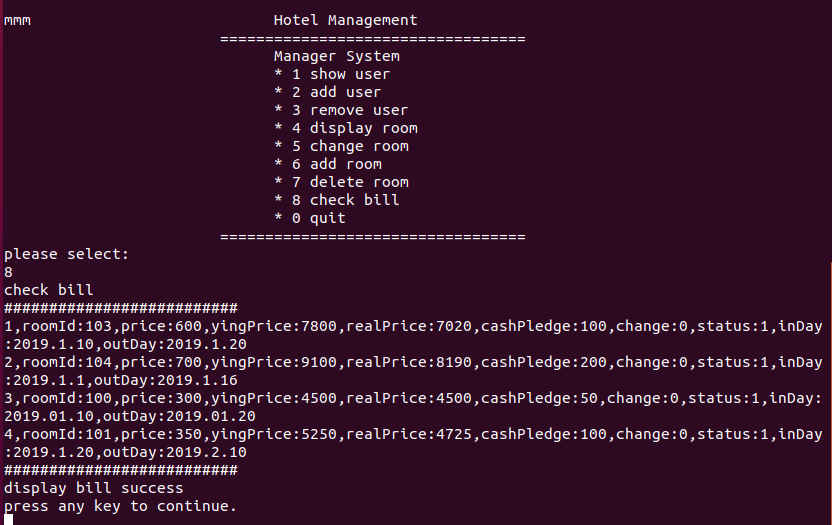完整代码链接：https://gitee.com/hhb845531681/hotel-management.git


展开全文• 注：需要的源代码的网友们加我 QQ：2645144662 或 点击资源下载源代码， 谢谢！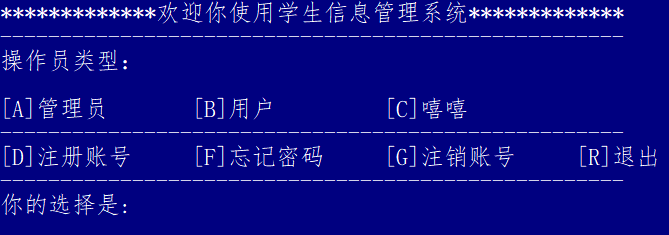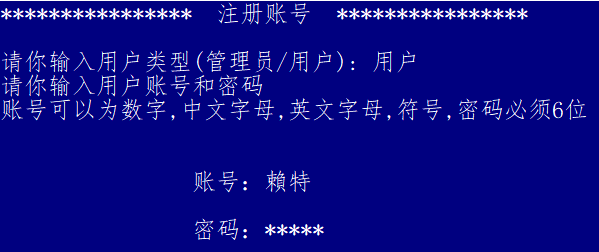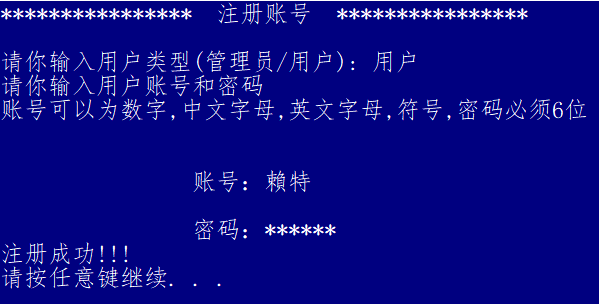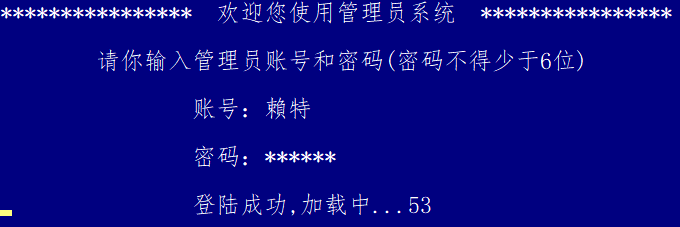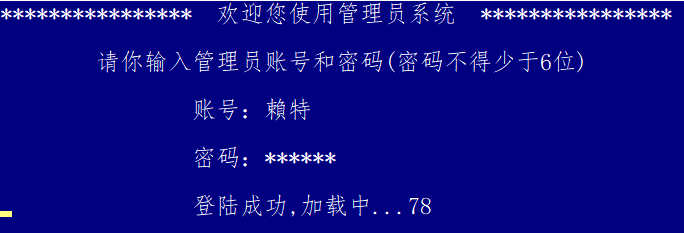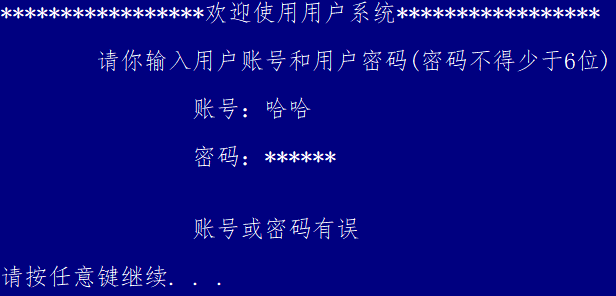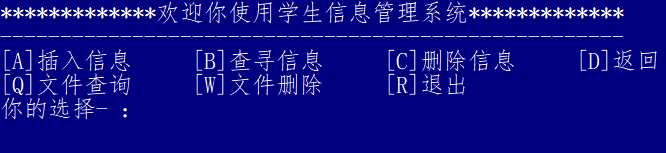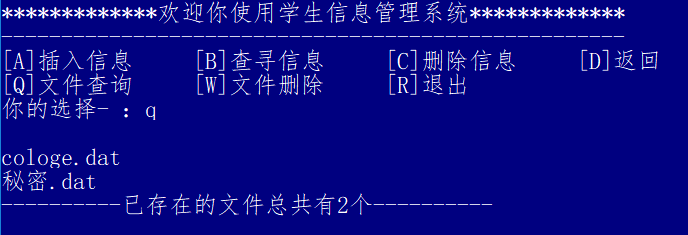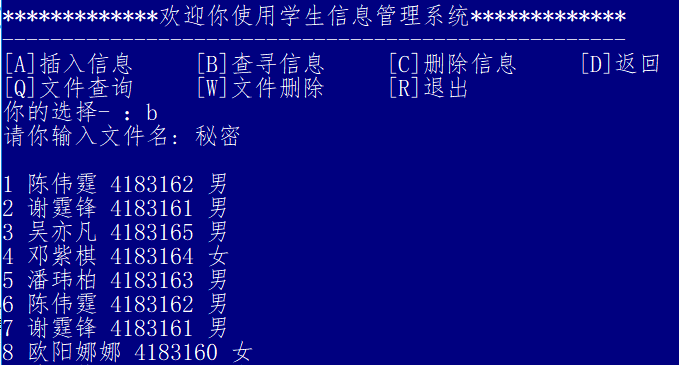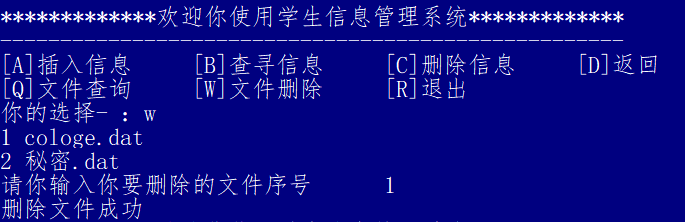注：需要的源代码的网友们点击资源下载源代码， 谢谢！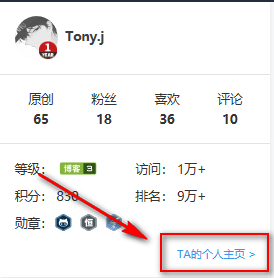源代码下载链接

提取码：gyy6

运行代码：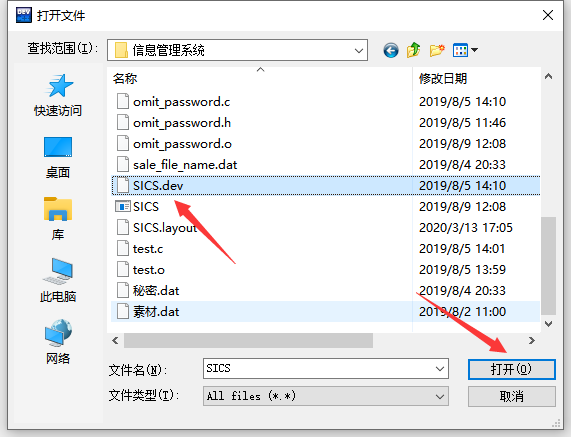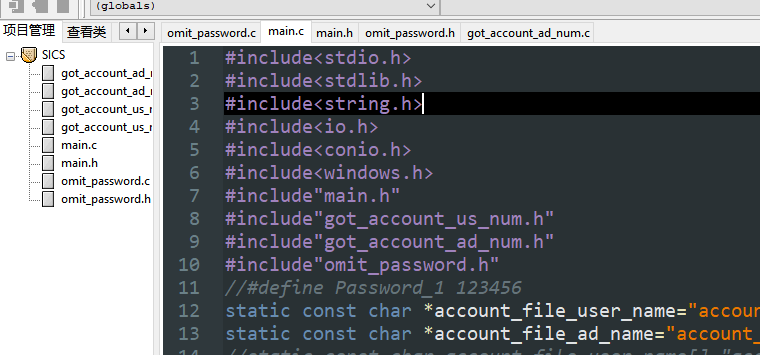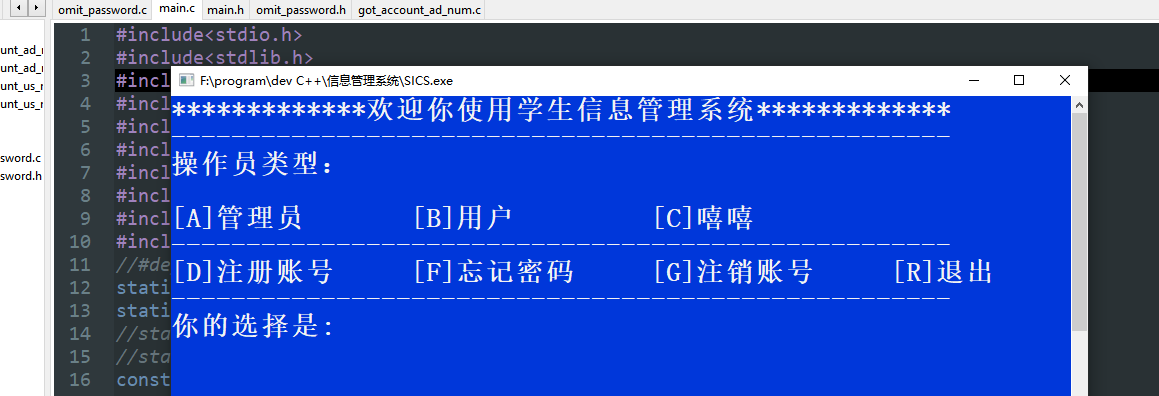展开全文• C语言实现简单地系统管理，对电影票的各种分类，时间，地点进行查找，寻找出符合要求的目标。
• 学生成绩管理系统 开发语言：C语言 开发工具：Visual Studio 2019 开发时间：2019.4.14 开发者：summer @一、系统使用展示 @二、系统功能 @三、菜单 @四、录入学生信息 @五、打印学生信息 @六、保存学生信息 @七、...链表
• c语言的数据结构和链表实现的销售管理系统。用两个c文件实现
• <span style="font-size:18px;">#include <stdio.h> #include <stdlib.h> #define FILENAME "student.dat" typedef enum{MAN, WOMAN} SEX; typedef struct tagStudent ... //学生...
• ## c语言实现的简单学生管理系统

万次阅读 多人点赞 2017-05-31 20:37:19
简单学生管理系统c语言花了一个月的时间，学习了链表，文件，多文件编程，然后就开始写学生管理系统，比较简单的那种，实现了增，添，改，查，多种排序，输入的时候的限定，成绩分析的功能。遇到的问题很多，也...
• ## C语言学生成绩管理系统源代码

万次阅读 多人点赞 2018-03-21 20:27:20
大学C语言实训课，C语言学生成绩管理系统。 #include<stdio.h> #include<string.h> #include<math.h> struct student { int num; char name; float pingshi; float shiyan; ...C语言成绩管理 管理系统
• ## java学生信息管理系统（附源码）

万次阅读 多人点赞 2016-07-14 16:36:30
一： 介绍 1：实现登录界面 2：实现主界面 有两种操作 一种是按钮操作 另外一种是SQL语句。 ... 3：连接SQL server 2008 数据库,后续我更新了，加了一个MySql 版本。在我的下载链接里面都有，里面也有使用说明...java 连接SQL数据库
• ## C语言学生管理系统增删改查

万次阅读 多人点赞 2018-09-17 20:02:02
本身意义可能不大，但是有很多学生再刚开始学习的时候，往往布置的大作业就是利用C语言实现简单的控制台管理系统，往往要求使用的结构是 在内存中用链表存储数据 从txt中读取信息，并可以删除写入 完成信息的展示...C
• ## c语言实现《学生管理系统》

千次阅读 多人点赞 2019-06-27 15:40:20
系统主要用于学校学生信息管理，总体任务是实现学生信息关系的系统化、科学化、规范化和自动化，其主要任务是用计算机对学生的各种信息进行日常管理，如查询、修改、增加、删除等。 功能介绍：    ...
• 大一上c语言学生管理系统* 你好！ 这是你第一次使用 c语言做完整的一个小程序，如果你想学习c语言不那么枯燥, 可以仔细阅读这篇文章，了解一下c语言的基本语法知识。 主要函数功能 1.打印功能菜单 2. 录入学生信息。...指针 函数闭包
• 一.题目分析(学生信息管理系统) 做一个学生信息管理系统，首先定义一个...在menu()方法中，用一个循环来一直输出这个学生管理系统的界面，直到输入8才结束 二.关键算法构造 N-S盒图： 流程图： 在这里插入图片描...
• 不论是在校计算机专业的学生还是刚步入IT行业从事C工作的毕业生，对于C语言的基础考核，学生管理系统则作为不二的选择。该项目不论从难易程度还是知识考核点都易于被接受。
• ## C语言链表实现学生管理系统

千次阅读 多人点赞 2019-06-19 21:38:02
C语言链表实现学生管理系统 #include "stdio.h" #include "stdlib.h" #include "string.h" struct Student { unsigned long ID; char Name; float Score; }; struct Node { struct ...
• int Menu(void) //兼容型菜单 {  int i;  system("title 学生成绩管理系统V4.0 Create By Lu Tong");  printf("\n\n");  printf(" ...
• 自己实现的C语言学生管理系统，带文本数据库，写了对文本文件的操作。 创建学生数据库（文件） 向数据库中录入内容 显示指定文件（数据库）中的内容 按照总分进行排序 向数据库中增加一条学生记录 删除一...
• 利用c语言实现了一个学生管理系统，能够完成学生数据管理系统的常用功能小项目
• 用指针的结构编写学生管理系统中的显示板块的子程序怎么写？谢谢！
• 简单的学生管理系统内存版，利用c语言编写 可以进行简单的增删改查
• #include<stdio.h> #include<stdlib.h> #define MAX_size 30 #define S struct student struct student { int num; char name; char sex; int age; }; void input(S s1[],int n) ......
• ## C语言图书管理系统设计报告

万次阅读 多人点赞 2017-06-20 17:37:54
源代码：https://blog.csdn.net/k_young1997/article/details/73480766 XXXX大学 ...C语言课程设计报告 ...题 目 图书管理系统设计 专业班级 XXXX级计算机科学与技术本科X班 组 别 计科第...
• 开发界面 链表知识 VC++6.o 文件读写 结构体
• 学生管理系统 C语言 通过运行（ 增删改查）学生管理系统 C语言 通过运行（ 增删改查）学生管理系统 C语言 通过运行（ 增删改查）
• ## java 学生信息管理系统

万次阅读 多人点赞 2015-10-06 14:03:33
学生信息管理包括添加，删除，修改，查询，显示全部等 具体结构如图 在SQL Server 2005数据库上实现数据操作。使用纯面向对象的java语言作为开发语言 在sql server 2005新建一个名为Student的数据库，在下面新建一......